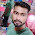### Program 80:To count number of words in a sentence

Program 80:

```#include<stdio.h>
#include<string.h>
main()
{
int i,word=1;
char str;
printf("Enter a string\n");
gets(str);
for(i=0;i<strlen(str);i++)
{
if(str[i]==' ')
{
word++;
}
}
printf("%d\n",word);

}```
Explanation:

1. This program starts with initializing :
• str → To store string with length of 100 which means it can store 100 letters
• i →Used as helping variable
• word→ To store number of words in a given string and is initilized to 1.
2. ```printf("Enter a string\n");
gets(str); ```
To take input from user
3. ```for(i=0;i<strlen(str);i++)
{```
it will traverse from i=0 to length of the string.For example if length of string is 10 for helloworld then it will iterate 10 times.From our example "I love Harry Potter" has length of 19 characters (remember to include space as it also counts as character) then for loop will iterate 19 times from 0 to 18.
4. ```if(str[i]==' ')
{
word++;
}```
Each time the loop iterates, each character is checked whether it is space or not as Number of spaces is equal to number of words if words count start from 1.

5. Ok.Lets take an example of I love harry potter.
• Iteration 1:- i=0;words=1 and strlen(str)=19 as 0<19 the loop is executed
• str[i]==' ' →str='I' which is not equal to ' '(i.e. space) so if part is not executed
• so word=1 remains same without any increment.
• final values after 1st iteration is i=1,word=1
• Iteration 2:- i=1;words=1 and strlen(str)=19 as 1<19 the loop is executed
• str[i]==' ' →str=' ' which is  equal to ' '(i.e. space) so if part is executed
• so word=1 is incremented by 1 so word=2.(*remember it is post increment but to avoid confusion I am showing that it will increment in this iteration  but the actual truth is it will be incremented in next iteration. To clearly understand post and pre increment refer its concept.).
• final values after 1st iteration is i=2,word=2
• Iteration 3:- i=2;words=2 and strlen(str)=19 as 2<19 the loop is executed
• str[i]==' ' →str='l' which is not equal to ' '(i.e. space) so if part is not executed
• so word=2 remains same without any increment.
• final values after 1st iteration is i=3,word=2
• Like this word will be incremented to 3 at 6th iteration and to 4 at 12th iteration.
• Finally word are 4

Output:

#### 1 comment:

1.college-party-guide One of the most important rituals for students in college is to throw the most memorable student dorm celebration.
factorial hundred In the last few days, the “factorial of 100” is one of the top subjects and a lot of maths geeks compute it using voice assistants such as Alexa, Shiri, etc.
factorial hundred In the last few days, the “factorial of 100” is one of the top subjects and a lot of maths geeks compute it using voice assistants such as Alexa, Shiri, etc.
gigolo The term”gigolo” usually refers to the person who lives an arranged lifestyle that includes many of these relationships in a series, rather than having any other support system.